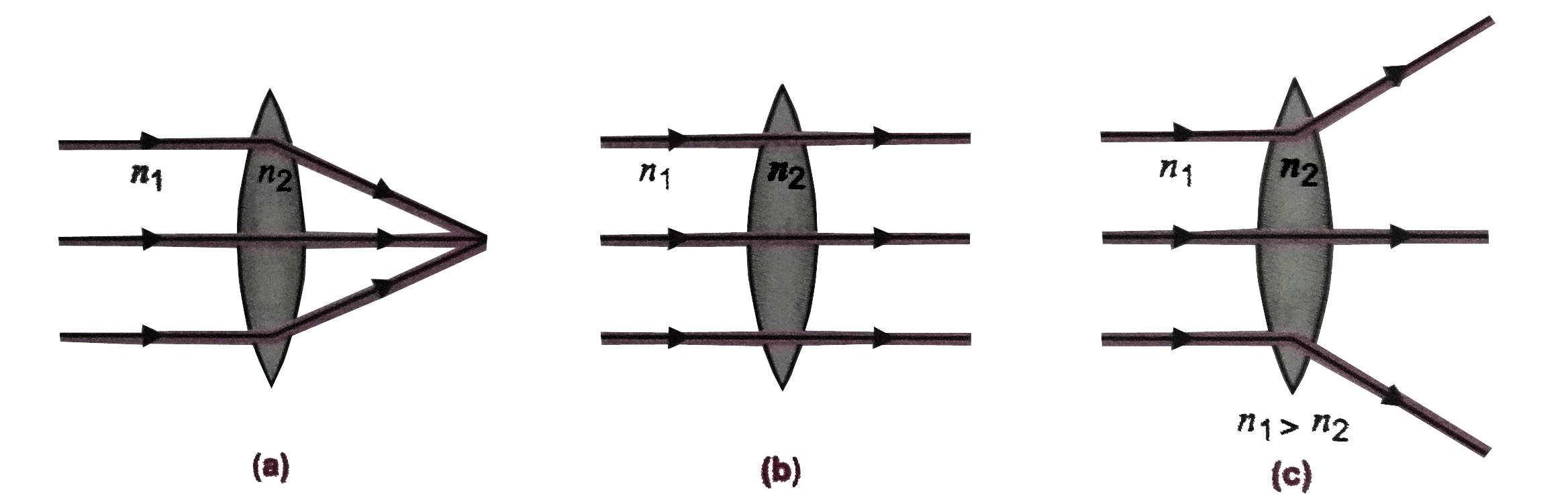# A convex lens made of a material of refractive index n_2 is kept in a medium of refractive index n_1. A parallel beam of light is incident on the

260 views
in Physics
closed
A convex lens made of a material of refractive index n_2 is kept in a medium of refractive index n_1. A parallel beam of light is incident on the lens. Complete the path of rays of light emerging from the convex lens if
(i) n_1 lt n_2 (ii) n_1 = n_2 (iii) n_1 gt n_2.

by (71.8k points)
selected by

When n_1 lt n_2, light goes from rarer to denser medium. Therefore, on passing through a convex lens, it converges,(ii) When n_1=n_2, there is no change in medium.Therefore, no bending or refraction occurs as shown in figure.
(iii) When n_1 gt n_2, light goes from denser to rarer medium. Therefore, no passing through a convex lens, it diverges as shown in figure.